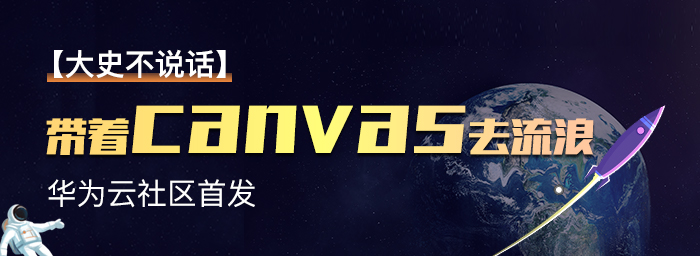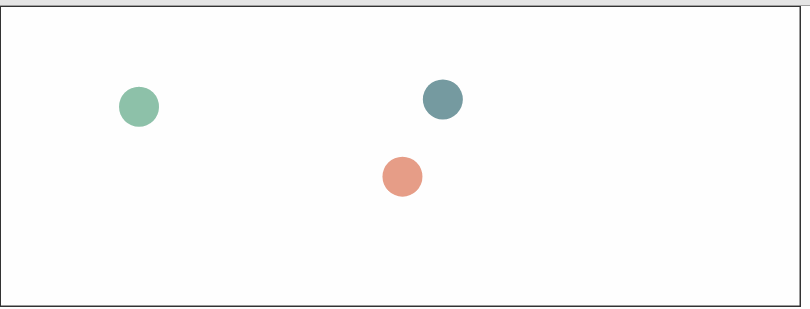# 【带着canvas去流浪（8）】碰撞## 二. 动画框架

canvas上实现基本的动画，可以遵循一个基本的编程框架:

function step(){
/**
*在每一帧中要执行的逻辑
*......
*/
requestAnimationFrame(step);
}

step();//启动执行


let stage = [];
stage.push(background, tree, cloud, sun);

function step(){
cleanStage();//对画布进行必要擦除
background.update();//更新土地的属性
tree.update();//更新树的属性
cloud.update();//更新云的属性
sun.update();//更新太阳的属性（属性中必然包含着太阳的位置数据）
background.paint();//绘制土地
tree.paint();//绘制树
cloud.paint();//绘制云
sun.paint();//绘制太阳
requestAnimationFrame(step);
}


//建立舞台及添加元素的代码
let stage = [];
stage.push(background, tree, cloud, sun....);

//逐帧动画代码
function step(){
cleanStage();
stage.map(sprite=>{
sprite,update();
sprite.paint(ctx);
});
requestAnimationFrame(step);
}


## 三. 在canvas中模拟碰撞

### 3.1定义小球的属性

class Ball{
constructor(x,y,id){
this.pos = new Vector2(x,y);//初始化小球的位置
this.id = id;
this.color = '';//绘制的颜色
this.r = 20;//小球半径，为方便演示，此处使用给定值
this.velocity = null;//小球的速度
}
}


### 3.2 生成新的小球

//为全局balls数组增加一个新的小球,初始位置为(50,30),
let ball = new Ball(50,30,balls.length);
ball.color = colorPalette[parseInt(steps / 100,10) % 10];
ball.velocity = new Vector2(5*Math.random(), 5 * Math.random());
balls.push(ball);
}


if (steps % 100 === 0 && steps < 1500) {
}


step每循环100次（大约1.5秒）就会多生成一个向随机方向发射的小球，且小球的数量不能超过15个。

### 3.3 帧动画绘制函数step

step函数是动画的核心，我们需要在其中完成重绘背景，添加小球，更新每个小球，绘制小球这些逻辑（由于背景是静态的，示例中并没有将其抽象为精灵动画）。

function step() {
steps++;
//重绘背景
paintBg();
//每隔一定时间增加一个小球
if (steps % 100 === 0 && steps < 1500) {
}
//更新每个小球的状态
balls = balls.map((ball,index,originArr)=>{
ball.update(index,originArr);
ball.paint();//描线但不在画布上绘制
return ball;
});
//绘制每个小球位置
requestAnimationFrame(step);
}


### 3.4 定义小球的update方法

• 状态更新

状态更新一般包括自身状态更新和相对状态更新。自身状态的更新，比如你希望小球在运动过程中颜色会有变化，就属于自身状态的变化，相对状态变化一般指小球相对公共坐标系或某个参照对象而发生的宏观位置变化，比如本例中的小球位置变化。

• 碰撞检测

碰撞检测一般包括精灵是否与其他精灵发生碰撞，并需要对碰撞后造成的影响进行仿真。

/*更新状态

update(index,balls){

let nextPos;//模拟下一次落点

//1.计算下一次落点

//2.判断新位置是否碰触边界，如果是则边界法向的速度反向，假设碰撞过程是无能量损失
if (nextPos.x + this.r > rightBorder || nextPos.x < this.r) {
this.velocity.x = -1 * this.velocity.x;//速度分量反向
nextPos = this.pos;//取消当前帧的位置更新
}
if (nextPos.y + this.r > bottomBorder || nextPos.y < this.r) {
this.velocity.y = -1 * this.velocity.y;
nextPos = this.pos;
}

//3.判断是否与其他小球产生碰撞，为避免重复，每个小球只和比自己id更大的小球做检测
balls.map(ball=>{
if (ball.id > index && this.checkCollision(ball)) {
this.handleCollision(ball);
}
return ball;
});

//4.确认更新位置
this.pos = nextPos;
}


### 3.5 碰撞检测

//碰撞检测
checkCollision(ball){
return this.pos.subtract(ball.pos).length() < this.r + ball.r;
}


### 3.6 碰撞仿真

//处理碰撞
handleCollision(ball){
let ballToThis = this.pos.subtract(ball.pos).normalize();
let thisToBall = ballToThis.negate();
this.velocity = ballToThis.multiply(Math.abs(ball.velocity.length()*(ball.velocity.dot(ballToThis) / ball.velocity.length())));
ball.velocity = thisToBall.multiply(Math.abs(this.velocity.length()*(this.velocity.dot(ballToThis) / this.velocity.length())));
}


## 四. 下一步

posted @ 2019-04-22 21:06  大史不说话  阅读(564)  评论(0编辑  收藏